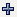Publication date: 05/24/2021

## Basic Column Properties

Basic column properties that apply generally include Formula and Notes.

### Formula

Insert a formula into a column to compute the values for that column. After a formula is added, the column is locked so that its data values cannot be manually edited (preventing invalidation of the formula).

Click Edit Formula to create a formula. For more information about creating a formula, see Create Formulas in JMP.

If you do not want JMP to evaluate the formula, click Suppress Eval.

If you do not want JMP to alert you about errors in your formula, click Ignore Errors.

Once you have created a formula:

In the Column Info window, a visual of the formula appears at right. However, if your formula is long, only a portion of it might appear. Click and drag the borders of the formula box to resize it.

From the data table, edit the formula by clicking () next to the column name in the Columns panel.

Tip: To bypass the Column Info window when creating a formula, right-click the column and select Formula.

### Notes

Adds notes to the selected column.